# What are Bioenergetics and What Are the Laws of Bioenergetics?

## What is Bioenergetics?

Bioenergetics which includes the two terms that is bio which means “within the living organism” and energetics means “production of energy”.

Bioenergetics is a field of biochemistry and cell biology that concerns with energy flow through the living systems. In a simpler way bioenergetics is defined as the study of energy transfer and utilization within the living organisms or biological systems.

Human requires the constant supply of energy to power all biological processes which also includes physical activity.

## Concept of Free Energy

Actual amount of energy which is available to do work is known as free energy

Change in Gibb’s free energy is equal to change in enthalpy minus temperature in Kelvin times change in entropy. Any processes with the negative Gibbs free energy is spontaneous which can be harness to perform the work.

The following equation expresses the changes in free energy in terms of both enthalpy and entropy.

ΔG =ΔH - TΔS

ΔG = Gibbs free energy expresses the amount of energy capable of doing work during the reaction at constant temperature and pressure which is a net driving force in a reaction.

ΔH = Enthalpy which is the heat content of the reacting system. It is the number and kinds of chemical bonds in the reactants and products.

ΔS = Entropy is the quantitative expression for the randomness in a system.

T = Absolute temperature

ΔG°= Standard free energy change at standard conditions i.e., at 298K/1atm pressure/1 molar concentration of reactants and products.

## Types of Bioenergetics Reactions

There are mainly 2 types of bioenergetics reactions. They are −

### Exergonic Reactions

These are spontaneous reactions and involve in the energy release without any utilization.

ΔG = GProducts – GReactants < 0 (Negative Value)

Example – Hydrolysis of ATP to ADP and phosphate is a form of exergonic reaction.

### Endergonic Reactions

These reactions are non-spontaneous and utilizes the free energy rather than releasing in order to complete the reaction. This can be expressed as

ΔG = GProducts – GReactants > 0 (Positive Value)

Example - Photosynthesis reaction which involves the synthesis of food utilizing carbon dioxide and the water in presence of light is a type of endergonic reaction.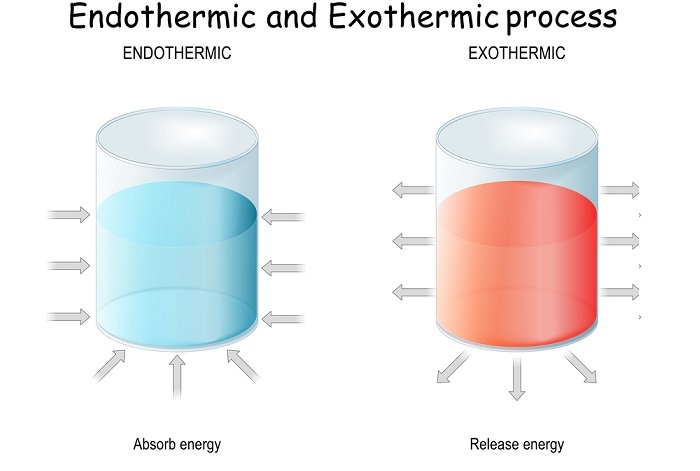## Importance of Bioenergetics

Bioenergetics is essential for carrying out following functions.

• Studying the bioenergetics helps in better understanding of the metabolism.

• Mechanical works like sitting, walking, talking etc.

• Chemical synthesis also requires energy.

• Growth and development.

• Cleavage or breaking of biomolecules within the body.

## What is Thermodynamics?

Understanding thermodynamics makes us easy to learn the concepts of bioenergetics. Thermodynamics is mainly the collection of laws and the principles which describes the flow of energy in a system. This system may be categorized into 3 different types.

• Isolated System: In an isolated system the energy or matter cannot be exchanged with the surroundings.

• Open System: Here both the energy and matter can be exchanged with the surroundings.

• Closed System: Here this system can exchange energy but not the matter with the surroundings.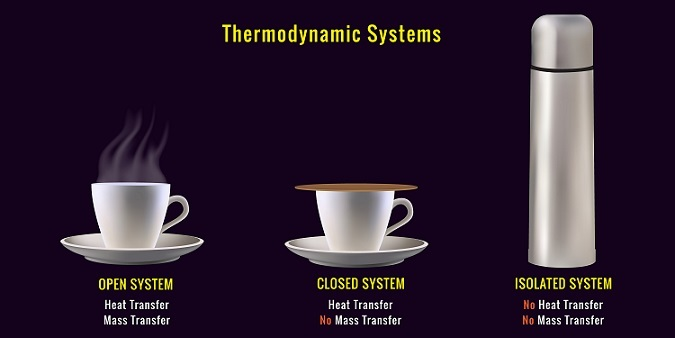## Laws of Bioenergetics: Thermodynamic laws

Laws of thermodynamics helps us to understand as why the energy flows in certain directions and ways.

### 1st Law of Thermodynamics

In concern with the changes in energy,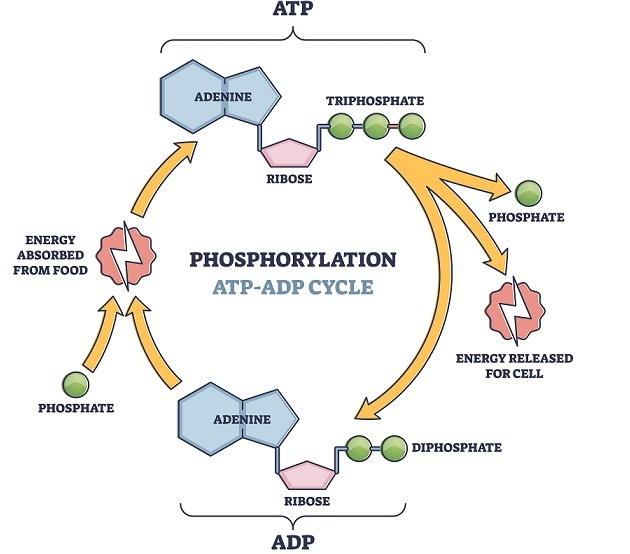according to this law energy cannot be created nor destroyed but instead it is shown to get transferred from one form of energy to another form that may be from potential energy to kinetic energy to heat energy etc. Here the total sum of energy of both the system and surroundings always remains constant unless there is any physical change or chemical reaction.

Example – In humans, energy is transferred through ATP and energy is stored in the 2 links of outer phosphate groups. The energy is released by the hydrolysis of ATP into ADP and inorganic phosphate and hydrogen ion. This released energy is used for muscle contraction during workout. However, ATP stored in muscle cells is limited so for the continuous supply of ATP to the body during exercise, our body activates 3 metabolic pathways. These are Phosphagen system, glycolytic system and oxidative system where all three synthesize ATP.

### 2nd Law of Thermodynamics

Here the concept of entropy comes into the role. This can be understood by the word disorder. Second law states that the entropy of a system and its surroundings must always increase. Within a system there is also a tendency to go towards the higher entropy. Here the correlation between the both forms of energy can be stated by this law.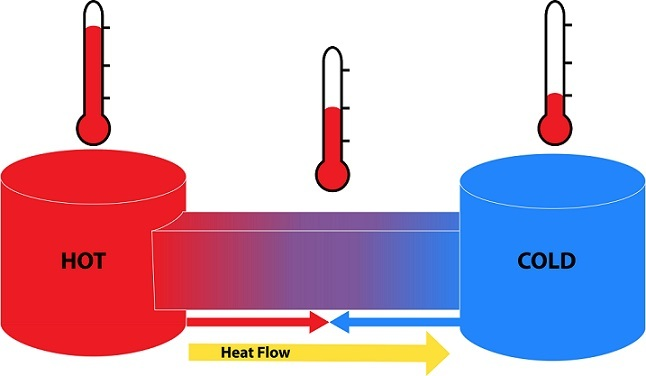Example – 1. Solid state of water is more ordered and has greater in entropy and liquid state is disordered and less in entropy. 2. Heat energy from a hot coffee cup flows to the solid surface.

### 3rd law of Thermodynamics

This law states that at an absolute zero the perfect crystalline solid molecule has zero entropy as this is the most ordered state a substance can be in. Entropy is measured in joules per kelvin and not a measure of energy but it is the distribution of energy within a system.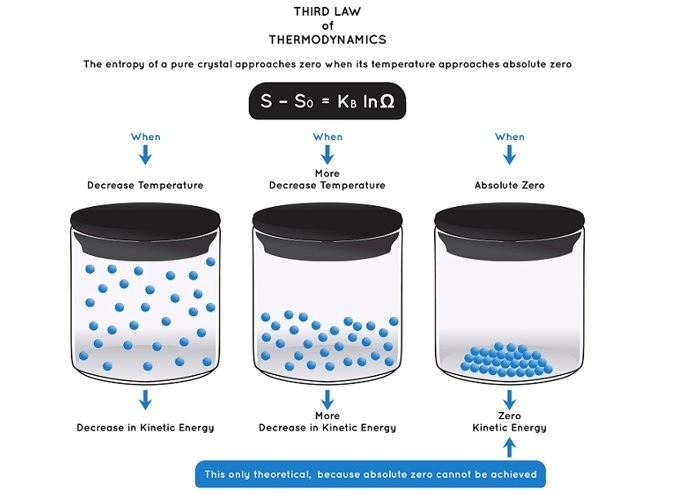## Conclusion

Bioenergetics is the study of biochemistry and deals with how the organisms manage the energy sources which is adenosine triphosphate (ATP) either by storing or producing or consuming. The change in free energy which is the energy to do work is always expressed in terms of change in enthalpy, temperature and change in entropy.

Enthalpy is the energy within the system and entropy is the distribution of energy in a system. If the change in free energy is negative, then the process is said to be spontaneous and when it is positive the process is non-spontaneous. One must know the thermodynamic laws to understand the flow of energy within a system. There are mainly 3 thermodynamic laws. These laws are important to understand the metabolism through the bioenergetics in humans.

Updated on: 11-May-2023

581 Views